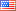# Revision history [back]

### Solving non linear system of equations

Why this is not working in sage?

f=-V+exp(V)-w-1; g=0.5*V-w; sol=solve(f==0,g==0],V,w,solution_dict=True)

It does not return numerical solutions when there are two.

Thanks2 No.2 RevisionShashank1887 ●24 ●46 ●80

### Solving non linear system of equations

Why this is not working in sage?

f=-V+exp(V)-w-1;
g=0.5*V-w;
sol=solve(f==0,g==0],V,w,solution_dict=True)sol=solve(f==0,g==0],V,w,solution_dict=True)


It does not return numerical solutions when there are two.

Thanks3 formattingShashank1887 ●24 ●46 ●80

### Solving non linear system of equations

Why this is not working in sage?

f=-V+exp(V)-w-1;
g=0.5*V-w;
sol=solve(f==0,g==0],V,w,solution_dict=True)
sol=solve([f==0,g==0],V,w,solution_dict=True)


It does not return numerical solutions when there are two.

Thanks